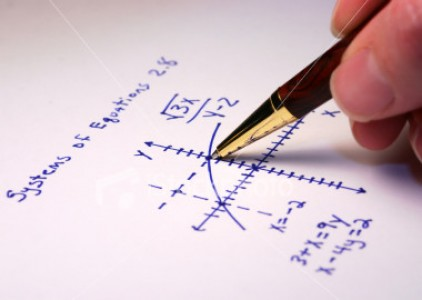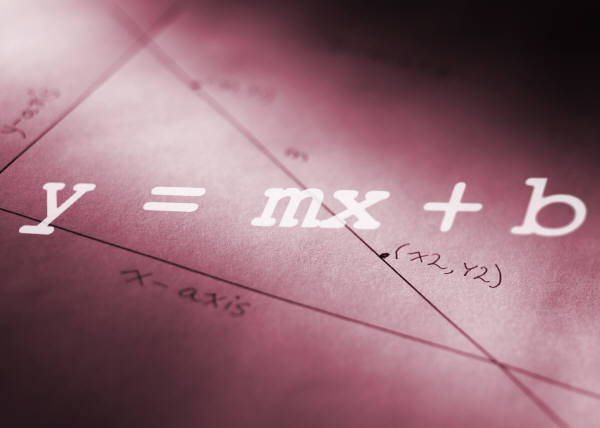# Difference Between Transitive and Substitution Property

Transitive and substitution property are two basic properties of mathematics that are used to solve mathematical expressions. Both these properties have their applications in algebra, and geometry as well. Substitution property, as the name suggests, is used to substitute values for various variables. It states that if x=y, then in an algebraic expression, we can easily replace every x by y, without affecting the solution. Transitive property, on the other hand, is used to define the equivalence relation between two and more variables. The transitive property of equality in algebra states that if a=b and b=c, then a=c.

Through definition, the transitive property looks similar to substitution property, where a third value c can be substituted for either of a or b. However, it is different from the former in the sense that the substitution property requires at least two values for comparison, whereas in transitive property three terms are compared.

### Instructions

• 1

Transitive Property

The transitive property of equality is defined as, “Let a, b and c be any three elements in set A, such that a=b and b=c, then a=c”.

In geometry, transitive property, for any three geometrical measurements, sides or angles, is defined as, “If two segments (or angles) are each congruent with a third segment (or angle), then they are congruent with each other”.

Image courtesy: cms.uhd.edu• 2

Substitution Property

The substitution property of equality states that “for any real numbers “a” and “b” if a = b then a can be substituted for b”. In simple words, a=b implies that b=a, therefore in any algebraic expression or equation, we can replace any ‘a’ with ‘b’ or any ‘b’ with ‘a’.

For example, if you are asked to solve an algebraic expression (x+7)/2 and it is given that x=5, then solve the expression by substituting the value of x. By putting x=5 in the above expression you get (5+7)/2 which is equal to 12/2, which equals 6. So, according to substitution property, if two values are equal to one another, they can be comfortably substituted for each other.

Besides algebra, substitution property is also used in geometry. When used in geometry, substation property of equality states that “if two geometric measurements are congruent then they can be easily replaced with one another in a statement involving one of them”.

These geometric objects can be two angles, two line segments, triangles etc. Essentially, the substitution property of equality in geometry says that if two things are equal in magnitude, it does not matter which one you use.

Image courtesy: cliffmass.blogspot.com•